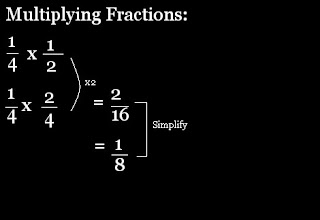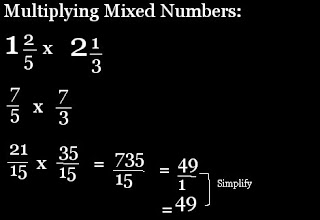### Sutchai's Last Fraction Post

Monday, May 11, 2009Adding Fractions:
1. Find common denominator.
2. Add both numerators and then add both denominators.
3. Simplify if it's possible.Adding Mixed Numbers:
1. Convert mixed numbers to improper fractions.
2. Find common denominator.
3. Add both numerators and then add both denominators.
4. Convert improper fraction to mixed number.
5. Simplify if it's possible.Subtracting Fractions:
1. Find common denominators.
2. Subtract numerators and leave the denominator as it is.
3. Simplify if it's possible.Multiplying Fractions:
1. Find common denominator.
2. Multiply both numerators and then multiply both denominators.
3. Simplify if it's possible.Multiplying Mixed Numbers:
1. Convert mixed numbers to improper fractions.
2. Find common denominator.
3. Multiply both numerators and then multiply both denominators.
4. Simplify if it's possible.Dividing Fractions:
1. Find the reciprocal of one of the fractions.
2. Multiply both of the numerators and then multiply both of the denominators.
3. Simplify if it's possible.Dividing Mixed Numbers:
1. Convert mixed numbers to improper fractions.
2. Find the reciprocal of one of the fractions.
3. Multiply both numerators and then multiply both denominators.
4. Simplify if it's possible.